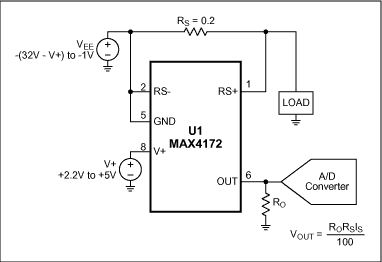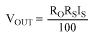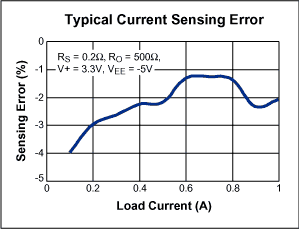# High-Side Positive Current Sensor Monitors Negative Supply

### Abstract

This application note uses a high-side current-sense amplifier to monitor the current from a negative supply voltage. The circuit uses the IC's wide common-mode input range to amplify a small Vsense signal at the negative supply and level shift it up to a positive output voltage.

### Introduction

All dedicated current-sense amplifiers are designed for high-side sensing on a positive supply, but you can adapt them to monitor a negative supply (Figure 1). The positive supply pin (V+) connects to the system's positive supply, and the ground pin (GND) connects to the negative supply (VEE). That arrangement monitors the negative supply while providing a positive output voltage for the external interface (typically an A/D converter). The RS+ pin of the current-sense amplifier (U1) connects to the load, and the RS- pin connects to the negative supply.U1's current-source output drives a current that is proportional to load current flowing to ground (not to the GND pin). Output resistor RO converts the current to a voltage, which is then digitized by an ADC (optional):The maximum output voltage is limited by saturation in the internal transistors (which occurs at approximately V+ -1.2V). Thus, V+ must exceed the full-scale output by at least 1.2V. If, for instance, the full-scale output is 1V, then V+ ≥ +2.2V. To meet the device's maximum and minimum operating voltages, 0 ≥ VEE ≥ -(32 - V+) and (V+ - VEE) ≥ +3V. Figure 2 shows the variation of current-measurement accuracy with load current.